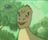cancel
Showing results for
Did you mean:

Fabric is Generally Available. Browse Fabric Presentations. Work towards your Fabric certification with the Cloud Skills Challenge.Helper I

## Help with DAX formula to calculate value from previous day

I need to create a calculated column 'Inventory_value' that calculates for each PV/product/date the inventory as follows:

The inventory of the day before for that PV/product + qty_em + qty_ref- qty_ven

If the inventory of the day before is not present, it becomes 0.

So far I've only made this and it doesn't work, because I always get a big value like as if its summing up all the rows together

```inventory_value =
var current_dt = rimanenze_pv_day_nonoil[dt]
var product = rimanenze_pv_day_nonoil[cost_prod]
var inventory_before =
CALCULATE(
SUM(rimanenze_pv_day_nonoil[em_total_value]),
FILTER(
rimanenze_pv_day_nonoil,
rimanenze_pv_day_nonoil[dt] = current_dt - 1
)
)

var inventory_true = inventory_before + rimanenze_pv_day_nonoil[em_qty] + rimanenze_pv_day_nonoil[rett_qty] - rimanenze_pv_day_nonoil[ven_qty]

var result =
IF(
ISBLANK(inventory_before),
0,
inventory_true
)
return

result```

The idea is that it returns that sum for each row, not all summed together. Does anyone have a clue on how to fix this? All the values are within the same table.

1 ACCEPTED SOLUTIONHelper I

Thank you, I used the additional detail as an AND measure like so

CALCULATE(
SUM(rimanenze_pv_day_nonoil[em_total_value]),
FILTER(
rimanenze_pv_day_nonoil,
rimanenze_pv_day_nonoil[dt] = current_dt - 1 && rimanenze_pv_day_nonoil[cost_prod]=product
)
)

I am not sure if this works, I will need to check thoroughly, but it does indeed give me more plausible results.
2 REPLIES 2Super User

you did not define the product?

```inventory_value =
var current_dt = rimanenze_pv_day_nonoil[dt]
var product = rimanenze_pv_day_nonoil[cost_prod]
var inventory_before =
CALCULATE(
SUM(rimanenze_pv_day_nonoil[em_total_value]),
FILTER(
rimanenze_pv_day_nonoil,        rimanenze_pv_day_nonoil[cost_prod]=product,
rimanenze_pv_day_nonoil[dt] = current_dt - 1
)
)

var inventory_true = inventory_before + rimanenze_pv_day_nonoil[em_qty] + rimanenze_pv_day_nonoil[rett_qty] - rimanenze_pv_day_nonoil[ven_qty]

var result =
IF(
ISBLANK(inventory_before),
0,
inventory_true
)
return

result```Helper I

Thank you, I used the additional detail as an AND measure like so

CALCULATE(
SUM(rimanenze_pv_day_nonoil[em_total_value]),
FILTER(
rimanenze_pv_day_nonoil,
rimanenze_pv_day_nonoil[dt] = current_dt - 1 && rimanenze_pv_day_nonoil[cost_prod]=product
)
)

I am not sure if this works, I will need to check thoroughly, but it does indeed give me more plausible results.Announcements#### Power BI Monthly Update - November 2023

Check out the November 2023 Power BI update to learn about new features.#### Fabric Community News unified experience

Read the latest Fabric Community announcements, including updates on Power BI, Synapse, Data Factory and Data Activator.#### The largest Power BI and Fabric virtual conference

130+ sessions, 130+ speakers, Product managers, MVPs, and experts. All about Power BI and Fabric. Attend online or watch the recordings.Top Solution Authors
Top Kudoed Authors
Users online (2,662)